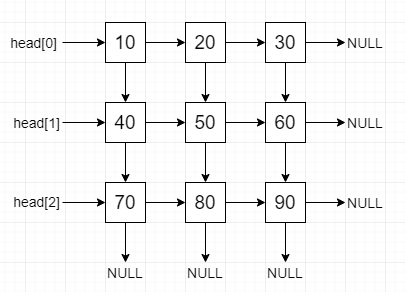# Construct a linked list from 2D matrix in C++

Suppose we have one matrix, we have to convert it to 2d linked list using recursive approach.

The list will have the right and down pointer.

So, if the input is like

 10 20 30 40 50 60 70 80 90

then the output will beTo solve this, we will follow these steps −

• Define a function make_2d_list(), this will take matrix mat, i, j, m, n,

• if i and j are not in the matrix boundary, then −

• return null

• temp := create a new node with value mat[i, j]

• right of temp := make_2d_list(mat, i, j + 1, m, n)

• down of temp := make_2d_list(mat, i + 1, j, m, n)

• return temp

## Example

Let us see the following implementation to get better understanding −

Live Demo

#include <bits/stdc++.h>
using namespace std;
class TreeNode {
public:
int data;
TreeNode *right, *down;
TreeNode(int d){
data = d;
right = down = NULL;
}
};
while (down_ptr) {
right_ptr = down_ptr;
while (right_ptr) {
cout << right_ptr->data << " ";
right_ptr = right_ptr->right;
}
cout << endl;
down_ptr = down_ptr->down;
}
}
TreeNode* make_2d_list(int mat[], int i, int j, int m, int n) {
if (i > n - 1 || j > m - 1)
return NULL;
TreeNode* temp = new TreeNode(mat[i][j]);
temp->right = make_2d_list(mat, i, j + 1, m, n);
temp->down = make_2d_list(mat, i + 1, j, m, n);
return temp;
}
int main() {
int m = 3, n = 3;
int mat[] = {
{ 10, 20, 30 },
{ 40, 50, 60 },
{ 70, 80, 90 } };
TreeNode* head = make_2d_list(mat, 0, 0, m, n);
}

## Input

{ { 10, 20, 30 },
{ 40, 50, 60 },
{ 70, 80, 90 } }

## Output

10 20 30
40 50 60
70 80 90